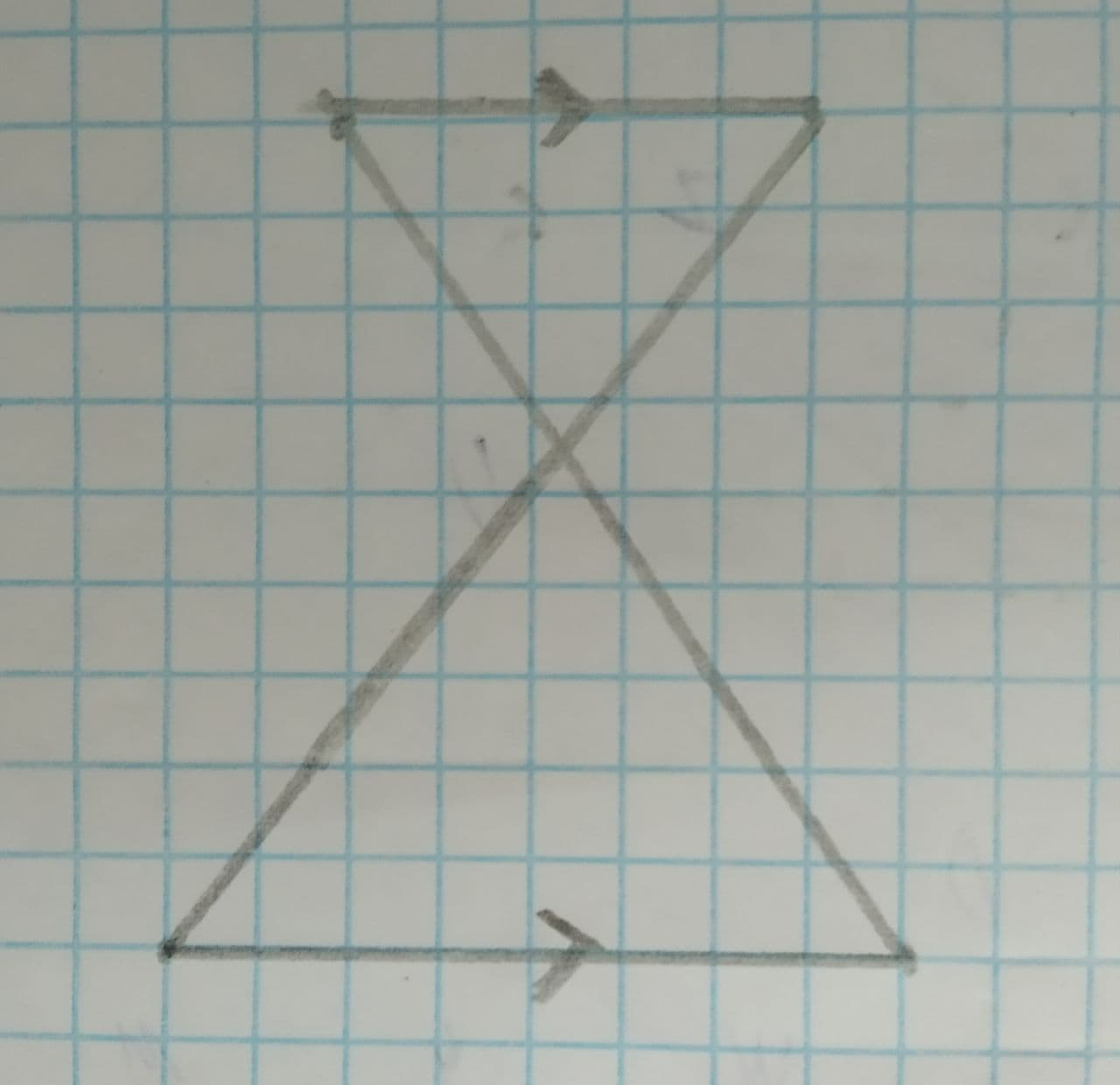# To prove the two triangles are similar using postulate or theorem.geduiwelh 2021-08-07 Answered
To prove: The two triangles are similar using postulate or theorem.
Given information:
The system triangles:• Questions are typically answered in as fast as 30 minutes

### Plainmath recommends

• Get a detailed answer even on the hardest topics.
• Ask an expert for a step-by-step guidance to learn to do it yourself.Szeteib

Now consider a figure, BNMLC in which two similar triangles are drawn such that they have a side parallel to the corresponding side.
$$\displaystyle\overline{{{B}{N}}}\le\overline{{{L}{C}}}$$
And when a line intersects two parallel lines, the corresponding angles of the intersections will be similar.
Therefore,
$$\displaystyle\angle{B}\stackrel{\sim}{=}\angle{C}$$
$$\displaystyle\angle{N}\stackrel{\sim}{=}\angle{L}$$
Thus, two angles of $$\displaystyle\triangle{B}{N}{M}$$ are congruent to two angles of $$\displaystyle\triangle{C}{L}{M}$$, therefore, according to the AA Similarity Postulate, the triangles are similar.
Therefore,
$$\displaystyle\triangle{B}{N}{M}\sim\triangle{C}{L}{M}$$
Hence, $$\displaystyle\triangle{B}{N}{M}\ {\quad\text{and}\quad}\ \triangle{C}{L}{M}$$ are similar with each other by the AA similarity theorem.